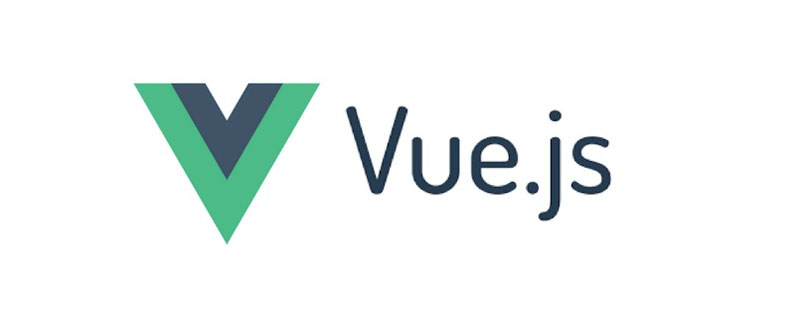# Vue.js 学习之三：与服务器的数据交互

Vue.js教程栏目今天介绍Vue.js学习之三的与服务器的数据交互。```const path = require('path');
const express = require('express')
const bodyParser = require('body-parser');
const knex = require('knex');
const port = 8080;

// 创建服务器实例
const app = express();

// 配置 public 目录，将其开放给浏览器端
app.use('/', express.static(path.join(__dirname, 'public')));
// 配置 node_modules 目录，将其开放给浏览器端
app.use('/node_modules', express.static(path.join(__dirname, 'node_modules')));

//配置 body-parser 中间件，以便获取 POST 请求数据。
app.use(bodyParser.urlencoded({ extended : false}));
app.use(bodyParser.json());

// 创建数据库连接对象：
const appDB = knex({
client: 'sqlite3', // 设置要连接的数据类型
connection: {      // 设置数据库的链接参数
filename: path.join(__dirname, 'data/database.sqlite')
},
debug: true,       // 设置是否开启 debug 模式，true 表示开启
pool: {            // 设置数据库连接池的大小，默认为{min: 2, max: 10}
min: 2,
max: 7
},
useNullAsDefault: true
});

appDB.schema.hasTable('notes')  // 查看数据库中是否已经存在 notes 表
.then(function(exists) {
if(exists == false) { // 如果 notes 表不存在就创建它
appDB.schema.createTable('notes', function(table) {
// 创建 notes 表：
table.increments('uid').primary();// 将 uid 设置为自动增长的字段，并将其设为主键。
table.string('noteMessage');      // 将 notes 设置为字符串类型的字段。
});
}
})
.then(function() {
// 请求路由
// 设置网站首页
app.get('/', function(req, res) {
res.redirect('/index.htm');
});

// 响应前端获取数据的 GET 请求
app.get('/data/get', function(req, res) {
appDB('notes').select('*')
.then(function(data) {
console.log(data);
res.status(200).send(data);
}).catch(function() {
res.status(404).send('找不到相关数据');
});
});

// 响应前端删除数据的 POST 请求
app.post('/data/delete', function(req, res) {
appDB('notes').delete()
.where('uid', '=', req.body['uid'])
.catch(function() {
res.status(404).send('删除数据失败');
});
res.send(200);
});

// 响应前端添加数据的 POST 请求
console.log('post data');
appDB('notes').insert(
{
noteMessage : req.body['noteMessage']
}
).catch(function() {
res.status(404).send('添加数据失败');
});
res.send(200);
});

// 监听 8080 端口
app.listen(port, function(){
console.log(`访问 http://localhost:\${port}/，按 Ctrl+C 终止服务！`);
});
})
.catch(function() {
// 断开数据库连接，并销毁 appDB 对象
appDB.destroy();
});```

```<!DOCTYPE html>
<html lang="zh-cn">
<meta charset="UTF-8">
<meta name="viewport" content="width=device-width, initial-scale=1.0">
<meta http-equiv="X-UA-Compatible" content="ie=edge">
<script defer="defer" src="/node_modules/vue/dist/vue.js"></script>
<script defer="defer" src="/node_modules/axios/dist/axios.js"></script>
<script defer="defer" src="/js/main.js"></script>
<title>留言本</title>
<body>
<p id="app">
<h1>留言本</h1>
<p id="showNote" v-for="note in notes">
<span>{{ note.userName }} 说：{{ note.noteMessage }} </span>
<input type="button" value="删除" @click="remove(note.uid)">
</p>
<h2>请留言：</h2>
<br>
<label :for="Message">写留言：</label>
<input type="text" v-model="Message"></input>
</p>
</p>
</body>
</html>```

```// 程序名称： Message
// 实现目标：
//   1. 学习 axios 库的使用
//   2. 掌握如何与服务器进行数据交互

const app = new Vue({
el: '#app',
data:{
Message: '',
notes: []
},
created: function() {
that = this;
axios.get('/data/get')
.then(function(res) {
that.notes = res.data;
})
.catch(function(err) {
console.error(err);
});
},
methods:{
if(this.userName !== '' && this.Message !== '') {
that = this;
noteMessage: that.Message
}).catch(function(err) {
console.error(err);
});
this.Message = '';
axios.get('/data/get')
.then(function(res) {
that.notes = res.data;
})
.catch(function(err) {
console.error(err);
});
}
},
remove: function(id) {
if(uid > 0) {
that = this;
axios.post('/data/delete', {
uid : id
}).catch(function(err) {
console.error(err);
});
axios.get('/data/get')
.then(function(res) {
that.notes = res.data;
})
.catch(function(err) {
console.error(err);
});
}
}
}
});```

• `el`成员：用于以 CSS 选择器的方式指定 Vue 实例所对应的元素容器，在这里，我指定的是`<p id="app">`元素。

• `data`成员：用于设置页面中绑定的数据，这里设置了以下三个数据变量：

• `notes`：这是一个数组变量，用于存放已被添加的留言记录。
• `userName`：这是一个字符串变量，用于获取"用户名"数据。
• `Message`：这是一个字符串变量，用于获取"留言"数据。
• `created`成员：用于在程序载入时做初始化操作，在这里，我从服务端读取了已被添加的留言记录，并将其加载到`notes`变量中。

• `methods`成员：用于定义页面中绑定的事件处理函数，这里定义了以下两个事件处理函数：

• `addNew`：用于添加新的留言记录，并同步更新`notes`中的数据。
• `remove`：用于删除指定的留言记录，并同步更新`notes`中的数据。

```<!-- 开发环境版本，包含了有帮助的命令行警告 -->
<script src="https://unpkg.com/axios/dist/axios.js"></script>
<!-- 或者 -->
<!-- 生产环境版本，优化了文件大小和载入速度 -->
<script src="https://unpkg.com/axios/dist/axios.min.js"></script>```### 全部评论我要评论

• 取消发布评论发送
• 1/1